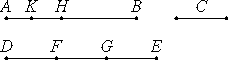Proposition 1

Two unequal magnitudes being set out, if from the greater there is subtracted a magnitude greater than its half, and from that which is left a magnitude greater than its half, and if this process is repeated continually, then there will be left some magnitude less than the lesser magnitude set out.

Let AB and C be two unequal magnitudes of which AB is the greater.

I say that, if from AB there is subtracted a magnitude greater than its half, and from that which is left a magnitude greater than its half, and if this process is repeated continually, then there will be left some magnitude which is less than the magnitude C.

cf. V.Def.4

Some multiple DE of C is greater than AB.

Divide DE into the parts DF, FG, and GE equal to C. From AB subtract BH greater than its half, and from AH subtract HK greater than its half, and repeat this process continually until the divisions in AB are equal in multitude with the divisions in DE.Let, then, AK, KH, and HB be divisions equal in multitude with DF, FG, and GE.

Now, since DE is greater than AB, and from DE there has been subtracted EG less than its half, and, from AB, BH greater than its half, therefore the remainder GD is greater than the remainder HA.

And, since GD is greater than HA, and there has been subtracted from GD the half GF, and from HA, HK greater than its half, therefore the remainder DF is greater than the remainder AK.

But DF equals C, therefore C is also greater than AK. Therefore AK is less than C.

Therefore there is left of the magnitude AB the magnitude AK which is less than the lesser magnitude set out, namely C.

Therefore, two unequal magnitudes being set out, if from the greater there is subtracted a magnitude greater than its half, and from that which is left a magnitude greater than its half, and if this process is repeated continually, then there will be left some magnitude less than the lesser magnitude set out.

Q.E.D.

And the theorem can similarly be proven even if the parts subtracted are halves.

Guide

The proof begins with two magnitudes C and AB and claims that some multiple of C is greater then AB. Definition V.Def.4 is not a justification for this statement. Euclid himself proved that a horn angle is less than any rectilinear angle in proposition III.16 and must have recognized that if the magnitude C is a horn angle, and the magnitude AB is a rectilinear angle, then no multiple of C is greater than AB. Nonetheless, he did not qualify this proposition to say that it only holds for certain kinds of magnitudes.

Use of this proposition

This proposition is the foundation of the method of exhaustion of Book XII. It is not used in the rest of Book X and would, perhaps, be better placed at the beginning of Book XII. This method is used in the propositions concerning areas of circles and volumes of solids. It is specifically used in propositions XII.2, XII.5, XII.10, XII.11, XII.12, and XII.16.